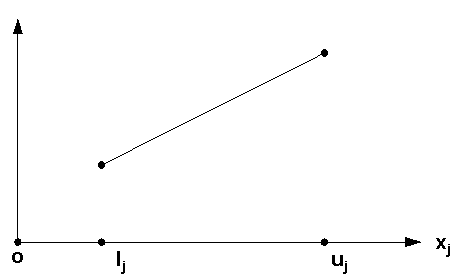# semi-continuous variables

Semi-continuous variables are variables that must take a value between their minimum and maximum or zero. So these variables are treated the same as regular variables, except that a value of zero is also accepted, even if there is a minimum bigger than zero is set on the variable.The practical usage of such variables is the following. If the variable is taken then take at least the minimum or else don't take it at all. For example in a blending problem this could mean if an amount of a raw material is taken then it must be at least the minimum or else take none at all. This because less than the minimum cannot be weighted for example. If a fixed minimum would be set then it would not be allowed to not to use the raw material at all. However via semi-continuous variables it is possible to state this kind of restrictions.

Since release 4, lp_solve has supported semi-continuous variables via the API call set_semicont or in the mps format in the bounds section via the SC bound type. See mps-format. If there is no minimum set on a semi-continuous variable then the default minimum is 1. The SC bound type normally needs the maximum in field 4. However this value may be omitted. In that case an upper bound of infinity is taken.

Example:

```NAME
ROWS
N  r_0
L  r_1
G  r_2
G  r_3
G  r_4
COLUMNS
x1        r_0                 -1   r_1                  1
x1        r_2                  2   r_3                 -1
x2        r_0                 -2   r_1                  1
x2        r_2                 -1   r_3                  3
x3        r_0                  4   r_4                  1
x4        r_0                  3   r_4                  1
RHS
RHS       r_1                  5   r_4                0.5
BOUNDS
SC BND       x3                  10
LO BND       x3                 1.1
ENDATA
```

The red lines are two lines that specify that variable x3 is a semi-continuous variable with a maximum of 10 and a minimum of 1.1. If the LO entry would not be there then the minimum would be 1, the default. If the 10 is omitted in the SC entry then there is no maximum.

Since lp_solve version 4.0.1.10, lp_solve also supports semi-continuous variables in the lp-format See lp-format. The above mps example would be in lp-format:

```max: x1 + 2x2 - 4x3 -3x4;
x1 + x2 <= 5;
2x1 - x2 >= 0;
-x1 + 3x2 >= 0;
x3 + x4 >= .5;
x3 >= 1.1;
x3 <= 10;

sec x3;
```

If the line x3 >= 1.1; is omitted then there is an implicit minimum of 1. If the line x3 <= 10; is omitted then there is no maximum on the variable. The new section sec is analogue as the int section and specifies all the variables that are semi-continuous. Multiple variables can be specified separated by a comma (,) or space.

The solution for this model is:

```Value of objective function: 6.83333

Actual values of the variables:
x1                   1.66667
x2                   3.33333
x3                   0
x4                   0.5
```

Why does it take 0 and not 1.1? Because the objective value is better if 0 is taken than 1.1. If the variable would not be semi-continuous (delete sec x3;) then the solution would be:

```Value of objective function: 3.93333

Actual values of the variables:
x1                   1.66667
x2                   3.33333
x3                   1.1
x4                   0
```

6.83333 is a better solution than 3.93333

If the model is changed to:

```max: x1 + 2x2 - 0.1x3 -3x4;
x1 + x2 <= 5;
2x1 - x2 >= 0;
-x1 + 3x2 >= 0;
x3 + x4 >= .5;
x3 >= 1.1;
x3 <= 10;

sec x3;
```

Only the cost of x3 is changed here from -4 to -0.1. The solution of this model is:

```Value of objective function: 8.22333

Actual values of the variables:
x1                   1.66667
x2                   3.33333
x3                   1.1
x4                   0
```

Now the solver takes the 1.1 as value because this solution is better than if 0 would be taken. This can be proven with the following model:

```max: x1 + 2x2 - 1x3 -3x4;
x1 + x2 <= 5;
2x1 - x2 >= 0;
-x1 + 3x2 >= 0;
x3 + x4 >= .5;
x3 <= 0;

sec x3;
```

This gives as solution:

```Value of objective function: 6.83333

Actual values of the variables:
x1                   1.66667
x2                   3.33333
x3                   0
x4                   0.5
```

6.83333 is worse than 8.22333, thus the solver takes the 1.1 solution.

Note that semi-continuous variables may be combined with integer variables. The same rules then apply, but the variable must also be integer then.

For example:

```max: x1 + 2x2 - .1x3 -3x4;
x1 + x2 <= 5;
2x1 - x2 >= 0;
-x1 + 3x2 >= 0;
x3 + x4 >= .5;
x3 >= 1.1;
x3 <= 10;

sec x3;

int x3;
```

The solution of this model is:

```Value of objective function: 8.13333

Actual values of the variables:
x1                   1.66667
x2                   3.33333
x3                   2
x4                   0
```

Because x3 must now be integer, it takes the value 2 instead of 1.1. The objective value 8.13333 is still better than he objective value if x3 would be 0 (6.83333).

In the mps format this can be either specified via the SI attribute or via SC attribute with the MARKER INTORG, MARKER INTEND in the COLUMNS section:

```ROWS
N  r_0
L  r_1
G  r_2
G  r_3
G  r_4
COLUMNS
x1        r_0                 -1   r_1                  1
x1        r_2                  2   r_3                 -1
x2        r_0                 -2   r_1                  1
x2        r_2                 -1   r_3                  3
x3        r_0                0.1   r_4                  1
x4        r_0                  3   r_4                  1
RHS
RHS       r_1                  5   r_4                0.5
BOUNDS
SI BND       x3                  10
LO BND       x3                 1.1
ENDATA
```

Or

```ROWS
N  r_0
L  r_1
G  r_2
G  r_3
G  r_4
COLUMNS
x1        r_0                 -1   r_1                  1
x1        r_2                  2   r_3                 -1
x2        r_0                 -2   r_1                  1
x2        r_2                 -1   r_3                  3
MARK0000  'MARKER'                 'INTORG'
x3        r_0                0.1   r_4                  1
MARK0001  'MARKER'                 'INTEND'
x4        r_0                  3   r_4                  1
RHS
RHS       r_1                  5   r_4                0.5
BOUNDS
SC BND       x3                  10
LO BND       x3                 1.1
ENDATA
```